# Damped response

Some images have been lost from this page during a recent server failure. We are working to restore the missing content.

In classical control theory, a second-order system has a transfer function like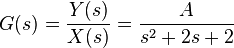$G(s) = \frac{Y(s)}{X(s)} = \frac{A}{s^2+2s+2}$

We can model a system like this fairly easily with ASCEND. First we need to reduce the model to first order differential equations: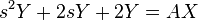$s^2 Y + 2 s Y + 2 Y = A X$

Now, let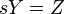$s Y = Z$ so that we have a pair of equations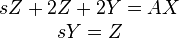$\begin{matrix} sZ + 2Z +2Y = AX \\ sY = Z \end{matrix}$

Taking the inverse Laplace transform and rearranging,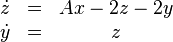$\begin{matrix} \dot{z} &=& Ax-2z -2y \\ \dot{y} &=& z \end{matrix}$

This can be coded into ASCEND in the usual syntax (see below).

Here is the output from the above system, with A = 2, x(t) = 1, plotted using OpenOffice. Different values of the coefficients in the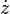$\dot{z}$ equation give different levels of damping, resonant frequency, etc.

Error creating thumbnail: File missing

This plot shows that the above transfer function has roots at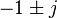$-1 \pm j$ as expected:

Here is the model that was used to produce these solutions:

REQUIRE "ivpsystem.a4l";

REQUIRE "atoms.a4l";
IMPORT "johnpye/extpy/extpy";

IMPORT "johnpye/roots";

(*
'Test problem'
We create a set of models with artificially-selected roots

and then check that the roots plot is as expected.
*)
MODEL simpleroots;

range IS_A set OF integer_constant;

range :== [7..8];
x[range], dx_dt[range] IS_A solver_var;

(* roots at -1 +/i j *)
dx_dt = 2.0 * 1.0 - 2.0 * x - 2.0 * x;

dx_dt = x;

t IS_A time;

METHODS
METHOD values;
x := 0;

x := 0;
END values;

METHOD ode_init;
FOR i IN [range] DO

x[i].ode_type := 1;
dx_dt[i].ode_type := 2;

x[i].ode_id := i;
dx_dt[i].ode_id := i;

x[i].obs_id := i;
END FOR;

t.ode_type := -1;
t := 0 {s};

END ode_init;

RUN default_self;

RUN reset; RUN values;
RUN ode_init;

RUN roots;